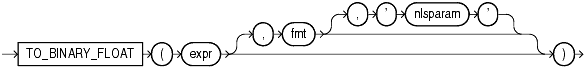## TO_BINARY_FLOAT

Syntax

to_binary_float::=Description of the illustration to_binary_float.gif

Purpose

`TO_BINARY_FLOAT` returns a single-precision floating-point number.

• `expr` can be a character string or a numeric value of type `NUMBER`, `BINARY_FLOAT`, or `BINARY_DOUBLE`. If `expr` is `BINARY_FLOAT`, then the function returns `expr`.

• The optional '`fmt`' and '`nlsparam`' arguments are valid only if `expr` is a character string. They serve the same purpose as for the `TO_CHAR` (number) function.

• The incase-sensitive string '`INF`' is converted to positive infinity.

• The incase-sensitive string '-`INF`' is converted to negative identity.

• The incase-sensitive string '`NaN`' is converted to `NaN` (not a number).

You cannot use a floating-point number format element (`F`, `f`, `D`, or `d`) in a character string `expr`.

Conversions from character strings or `NUMBER` to `BINARY_FLOAT` can be inexact, because the `NUMBER` and character types use decimal precision to represent the numeric value and `BINARY_FLOAT` uses binary precision.

Conversions from `BINARY_DOUBLE` to `BINARY_FLOAT` are inexact if the `BINARY_DOUBLE` value uses more bits of precision than supported by the `BINARY_FLOAT`.

Using table `float_point_demo` created for TO_BINARY_DOUBLE , the following example converts a value of datatype `NUMBER` to a value of datatype `BINARY_FLOAT`:
```SELECT dec_num, TO_BINARY_FLOAT(dec_num)